Скачать презентацию Public Key Cryptography and Cryptographic Hashes CS 461 ECE

81c8c1decffa13d5a9004d322b121c45.ppt

• Количество слайдов: 52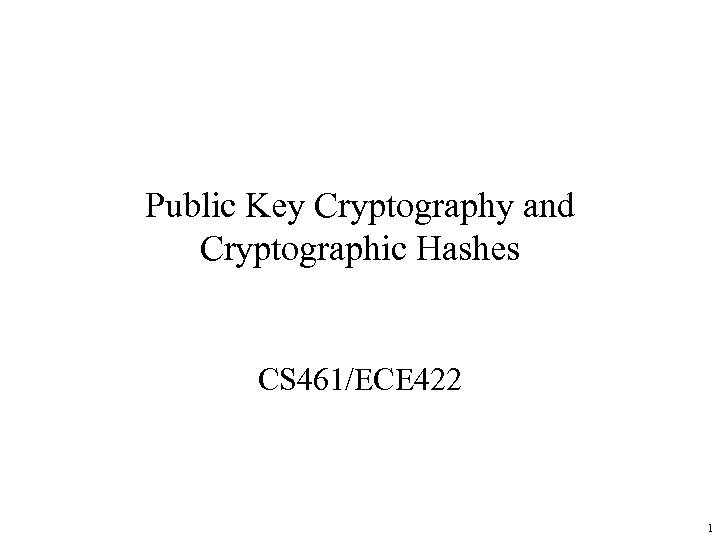Public Key Cryptography and Cryptographic Hashes CS 461/ECE 422 1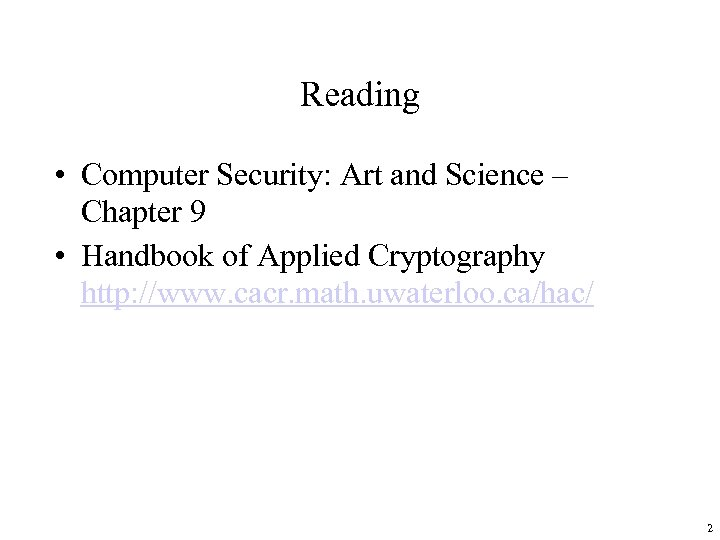Reading • Computer Security: Art and Science – Chapter 9 • Handbook of Applied Cryptography http: //www. cacr. math. uwaterloo. ca/hac/ 2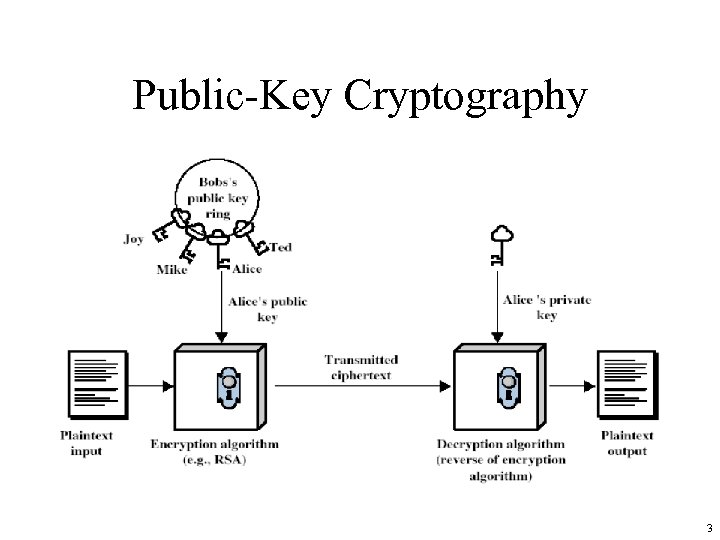Public-Key Cryptography 3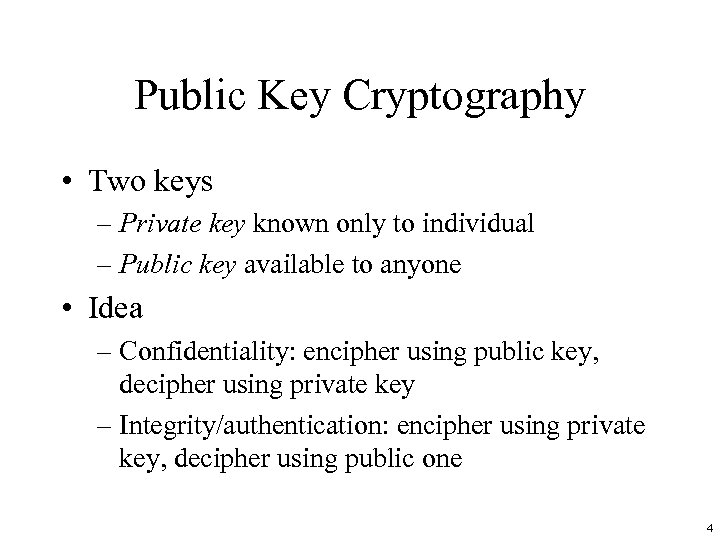Public Key Cryptography • Two keys – Private key known only to individual – Public key available to anyone • Idea – Confidentiality: encipher using public key, decipher using private key – Integrity/authentication: encipher using private key, decipher using public one 4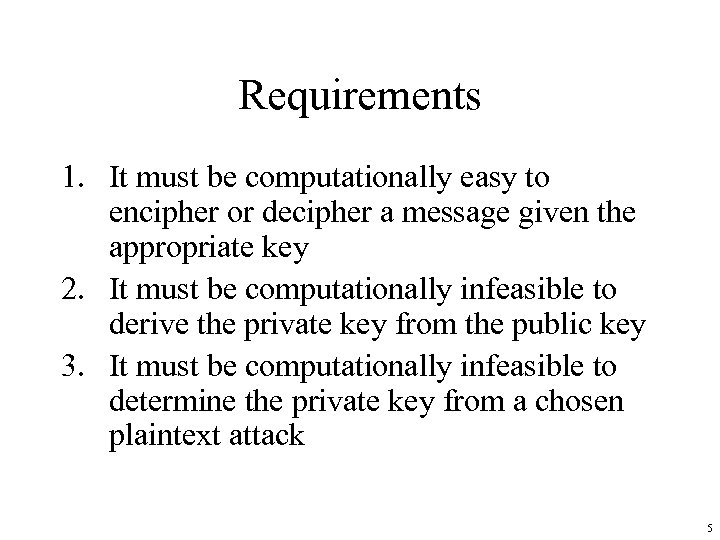Requirements 1. It must be computationally easy to encipher or decipher a message given the appropriate key 2. It must be computationally infeasible to derive the private key from the public key 3. It must be computationally infeasible to determine the private key from a chosen plaintext attack 5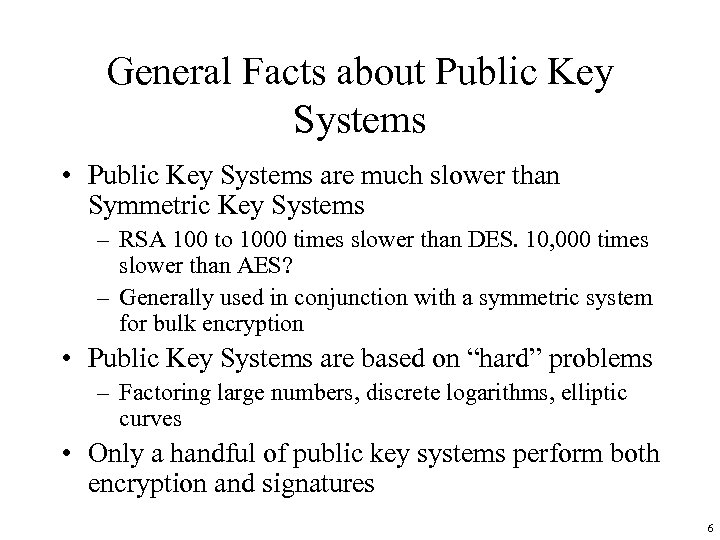General Facts about Public Key Systems • Public Key Systems are much slower than Symmetric Key Systems – RSA 100 to 1000 times slower than DES. 10, 000 times slower than AES? – Generally used in conjunction with a symmetric system for bulk encryption • Public Key Systems are based on “hard” problems – Factoring large numbers, discrete logarithms, elliptic curves • Only a handful of public key systems perform both encryption and signatures 6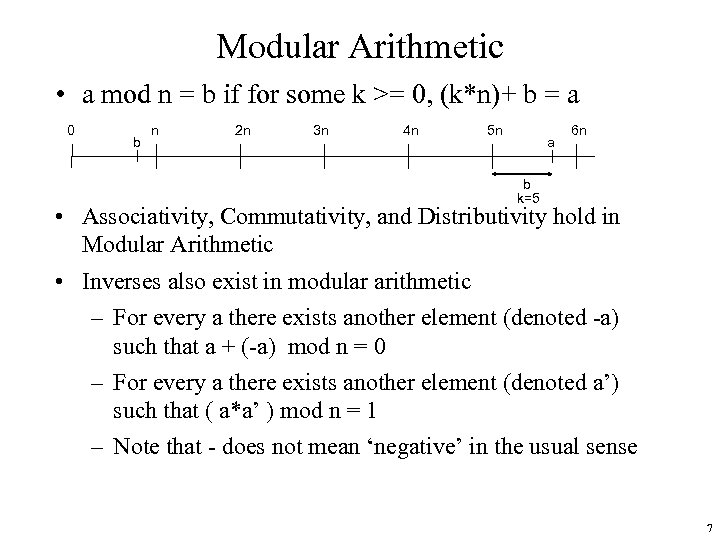Modular Arithmetic • a mod n = b if for some k >= 0, (k*n)+ b = a 0 b n 2 n 3 n 4 n 5 n a 6 n b k=5 • Associativity, Commutativity, and Distributivity hold in Modular Arithmetic • Inverses also exist in modular arithmetic – For every a there exists another element (denoted -a) such that a + (-a) mod n = 0 – For every a there exists another element (denoted a’) such that ( a*a’ ) mod n = 1 – Note that - does not mean ‘negative’ in the usual sense 7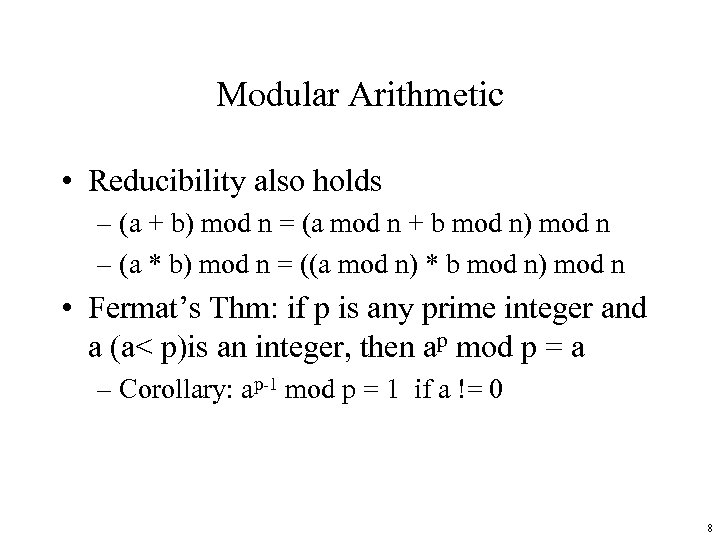Modular Arithmetic • Reducibility also holds – (a + b) mod n = (a mod n + b mod n) mod n – (a * b) mod n = ((a mod n) * b mod n) mod n • Fermat’s Thm: if p is any prime integer and a (a< p)is an integer, then ap mod p = a – Corollary: ap-1 mod p = 1 if a != 0 8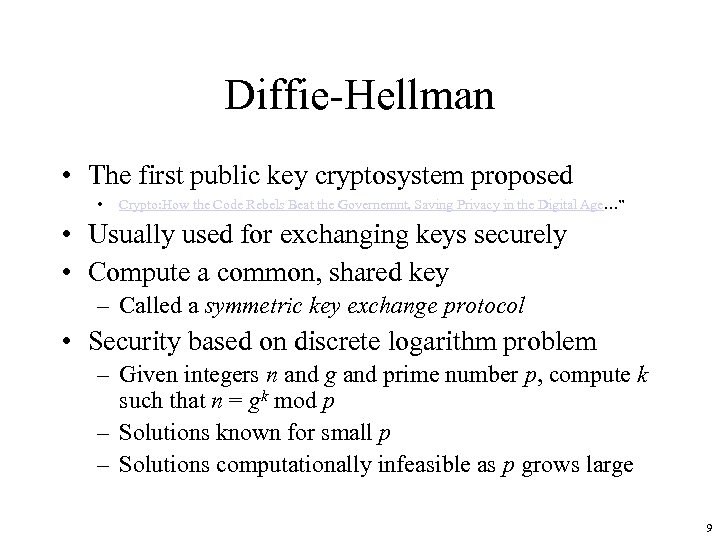Diffie-Hellman • The first public key cryptosystem proposed • Crypto: How the Code Rebels Beat the Governemnt, Saving Privacy in the Digital Age…” • Usually used for exchanging keys securely • Compute a common, shared key – Called a symmetric key exchange protocol • Security based on discrete logarithm problem – Given integers n and g and prime number p, compute k such that n = gk mod p – Solutions known for small p – Solutions computationally infeasible as p grows large 9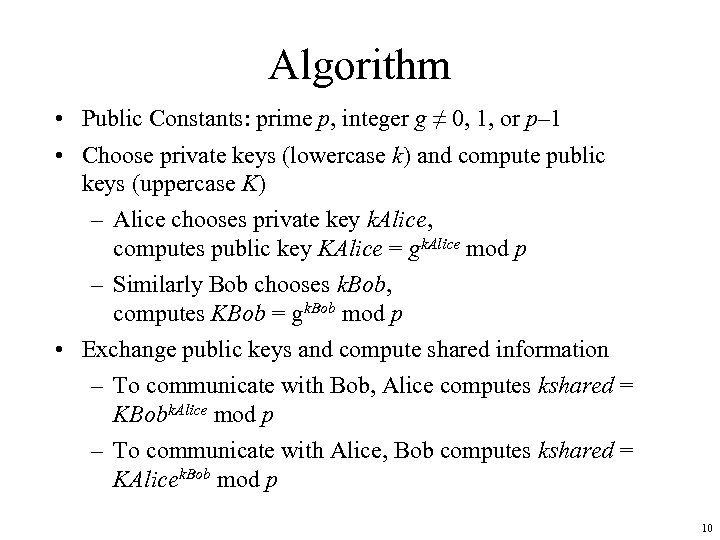Algorithm • Public Constants: prime p, integer g ≠ 0, 1, or p– 1 • Choose private keys (lowercase k) and compute public keys (uppercase K) – Alice chooses private key k. Alice, computes public key KAlice = gk. Alice mod p – Similarly Bob chooses k. Bob, computes KBob = gk. Bob mod p • Exchange public keys and compute shared information – To communicate with Bob, Alice computes kshared = KBobk. Alice mod p – To communicate with Alice, Bob computes kshared = KAlicek. Bob mod p 10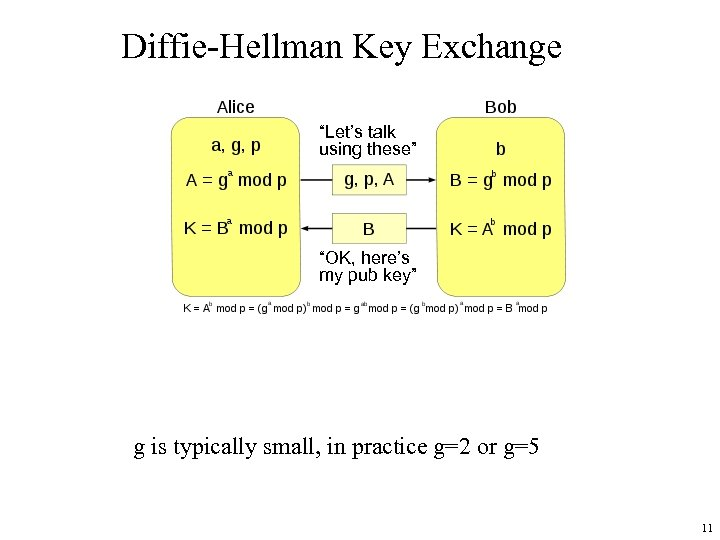Diffie-Hellman Key Exchange “Let’s talk using these” “OK, here’s my pub key” g is typically small, in practice g=2 or g=5 11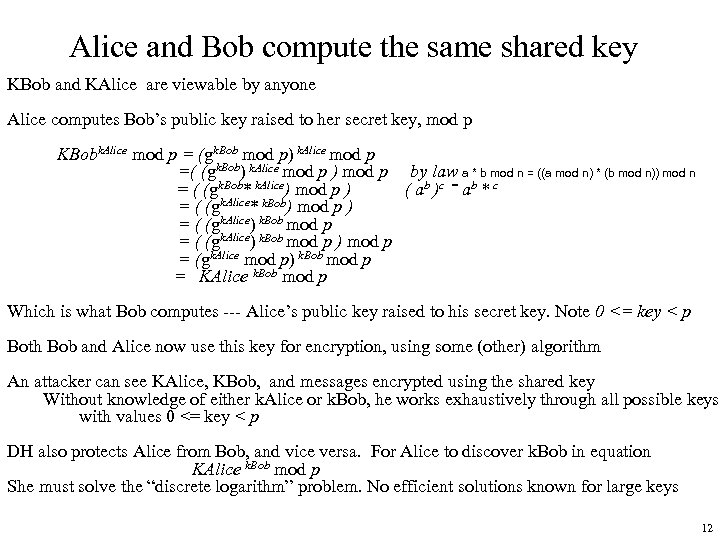Alice and Bob compute the same shared key KBob and KAlice are viewable by anyone Alice computes Bob’s public key raised to her secret key, mod p KBobk. Alice mod p = (gk. Bob mod p) k. Alice mod p =( (gk. Bob) k. Alice mod p ) mod p by law a * b mod n = ((a mod n) * (b mod n)) mod n = ( (gk. Bob* k. Alice) mod p ) ( ab )c = ab * c = ( (gk. Alice* k. Bob) mod p ) = ( (gk. Alice) k. Bob mod p ) mod p = (gk. Alice mod p) k. Bob mod p = KAlice k. Bob mod p Which is what Bob computes --- Alice’s public key raised to his secret key. Note 0 <= key < p Both Bob and Alice now use this key for encryption, using some (other) algorithm An attacker can see KAlice, KBob, and messages encrypted using the shared key Without knowledge of either k. Alice or k. Bob, he works exhaustively through all possible keys with values 0 <= key < p DH also protects Alice from Bob, and vice versa. For Alice to discover k. Bob in equation KAlice k. Bob mod p She must solve the “discrete logarithm” problem. No efficient solutions known for large keys 12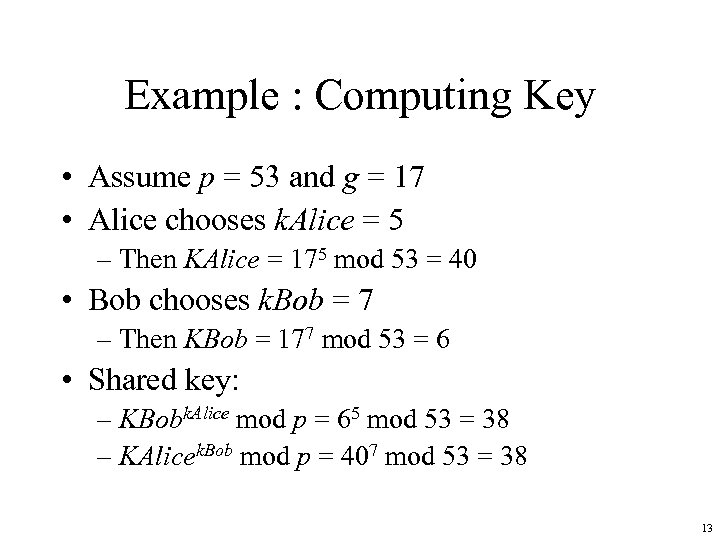Example : Computing Key • Assume p = 53 and g = 17 • Alice chooses k. Alice = 5 – Then KAlice = 175 mod 53 = 40 • Bob chooses k. Bob = 7 – Then KBob = 177 mod 53 = 6 • Shared key: – KBobk. Alice mod p = 65 mod 53 = 38 – KAlicek. Bob mod p = 407 mod 53 = 38 13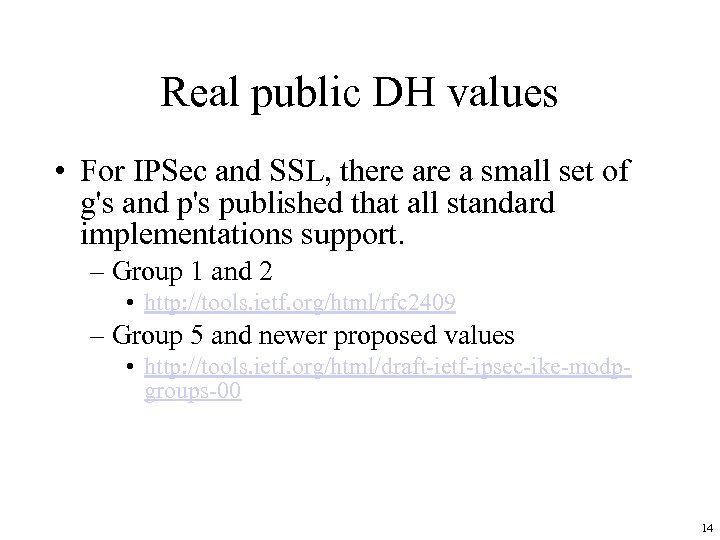Real public DH values • For IPSec and SSL, there a small set of g's and p's published that all standard implementations support. – Group 1 and 2 • http: //tools. ietf. org/html/rfc 2409 – Group 5 and newer proposed values • http: //tools. ietf. org/html/draft-ietf-ipsec-ike-modpgroups-00 14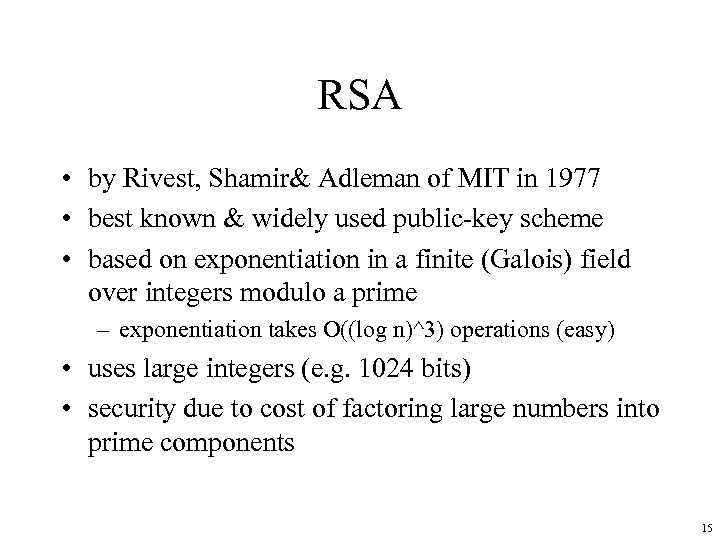RSA • by Rivest, Shamir& Adleman of MIT in 1977 • best known & widely used public-key scheme • based on exponentiation in a finite (Galois) field over integers modulo a prime – exponentiation takes O((log n)^3) operations (easy) • uses large integers (e. g. 1024 bits) • security due to cost of factoring large numbers into prime components 15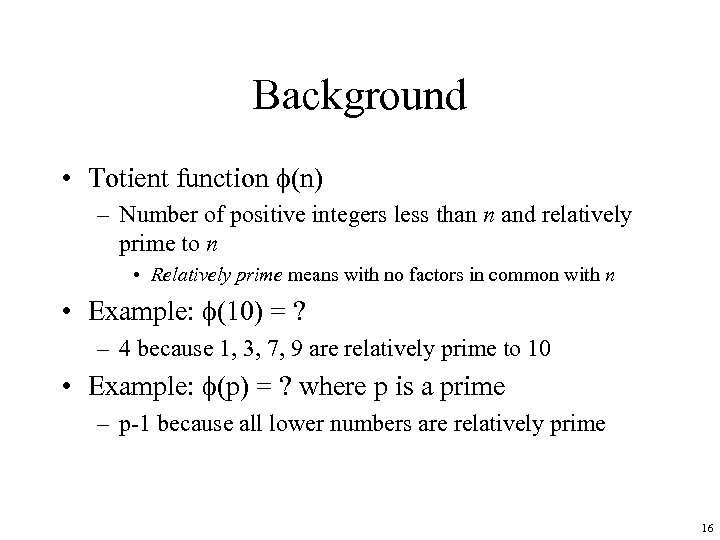Background • Totient function (n) – Number of positive integers less than n and relatively prime to n • Relatively prime means with no factors in common with n • Example: (10) = ? – 4 because 1, 3, 7, 9 are relatively prime to 10 • Example: (p) = ? where p is a prime – p-1 because all lower numbers are relatively prime 16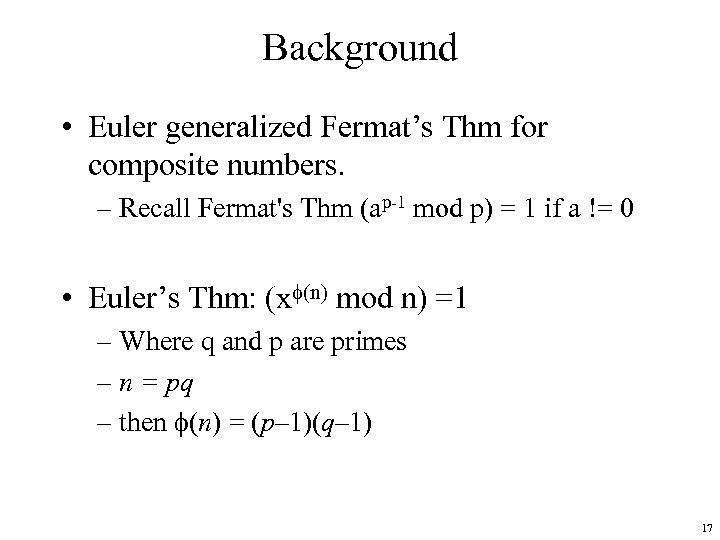Background • Euler generalized Fermat’s Thm for composite numbers. – Recall Fermat's Thm (ap-1 mod p) = 1 if a != 0 • Euler’s Thm: (x (n) mod n) =1 – Where q and p are primes – n = pq – then (n) = (p– 1)(q– 1) 17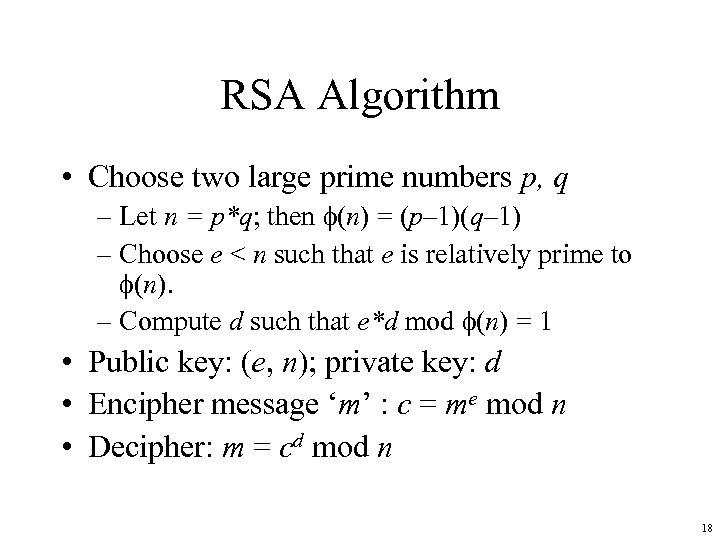RSA Algorithm • Choose two large prime numbers p, q – Let n = p*q; then (n) = (p– 1)(q– 1) – Choose e < n such that e is relatively prime to (n). – Compute d such that e*d mod (n) = 1 • Public key: (e, n); private key: d • Encipher message ‘m’ : c = me mod n • Decipher: m = cd mod n 18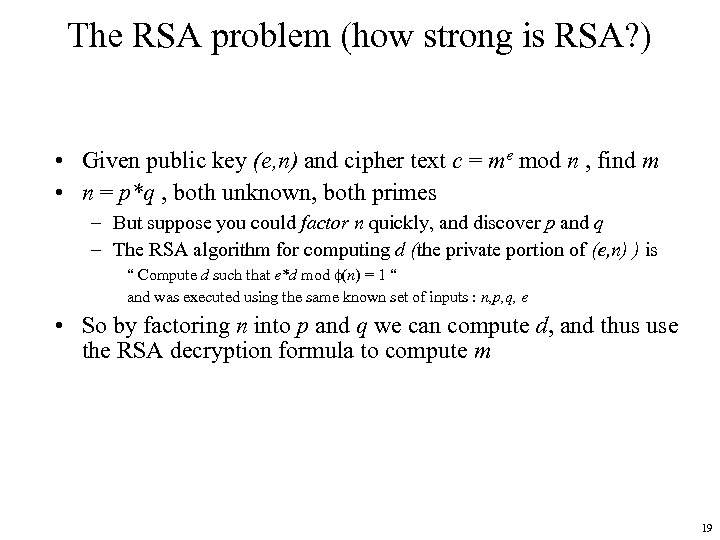The RSA problem (how strong is RSA? ) • Given public key (e, n) and cipher text c = me mod n , find m • n = p*q , both unknown, both primes – But suppose you could factor n quickly, and discover p and q – The RSA algorithm for computing d (the private portion of (e, n) ) is “ Compute d such that e*d mod (n) = 1 “ and was executed using the same known set of inputs : n, p, q, e • So by factoring n into p and q we can compute d, and thus use the RSA decryption formula to compute m 19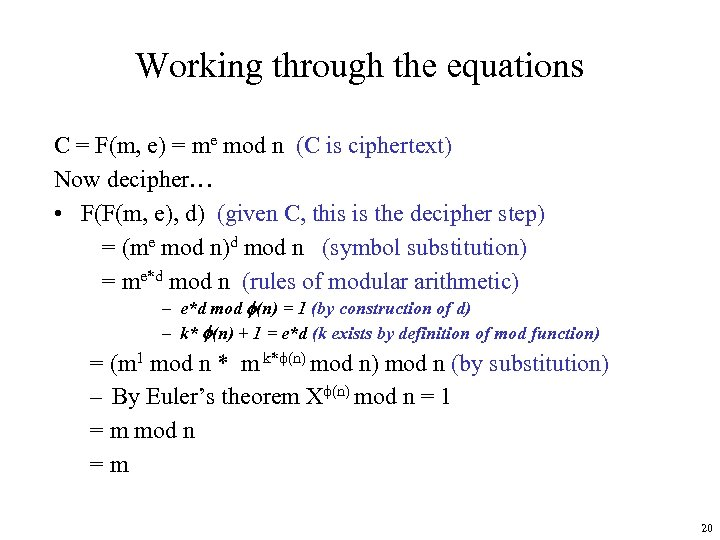Working through the equations C = F(m, e) = me mod n (C is ciphertext) Now decipher… • F(F(m, e), d) (given C, this is the decipher step) = (me mod n)d mod n (symbol substitution) = me*d mod n (rules of modular arithmetic) – e*d mod (n) = 1 (by construction of d) – k* (n) + 1 = e*d (k exists by definition of mod function) = (m 1 mod n * m k* (n) mod n (by substitution) – By Euler’s theorem X (n) mod n = 1 = m mod n =m 20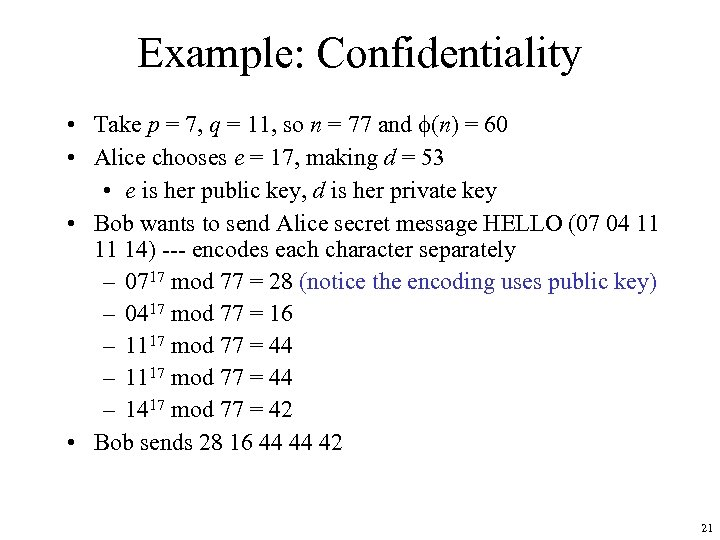Example: Confidentiality • Take p = 7, q = 11, so n = 77 and (n) = 60 • Alice chooses e = 17, making d = 53 • e is her public key, d is her private key • Bob wants to send Alice secret message HELLO (07 04 11 11 14) --- encodes each character separately – 0717 mod 77 = 28 (notice the encoding uses public key) – 0417 mod 77 = 16 – 1117 mod 77 = 44 – 1417 mod 77 = 42 • Bob sends 28 16 44 44 42 21Security Services • Confidentiality – Only the owner of the private key knows it, so text enciphered with public key cannot be read by anyone except the owner of the private key • Authentication – Only the owner of the private key knows it, so text enciphered with private key must have been generated by the owner 22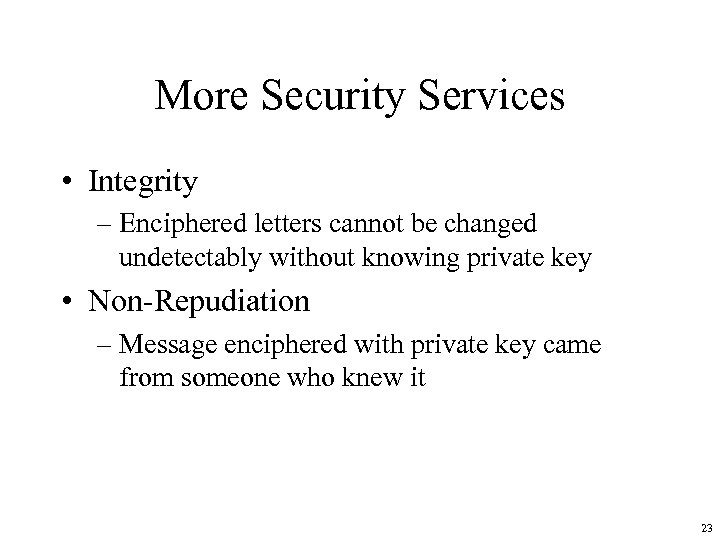More Security Services • Integrity – Enciphered letters cannot be changed undetectably without knowing private key • Non-Repudiation – Message enciphered with private key came from someone who knew it 23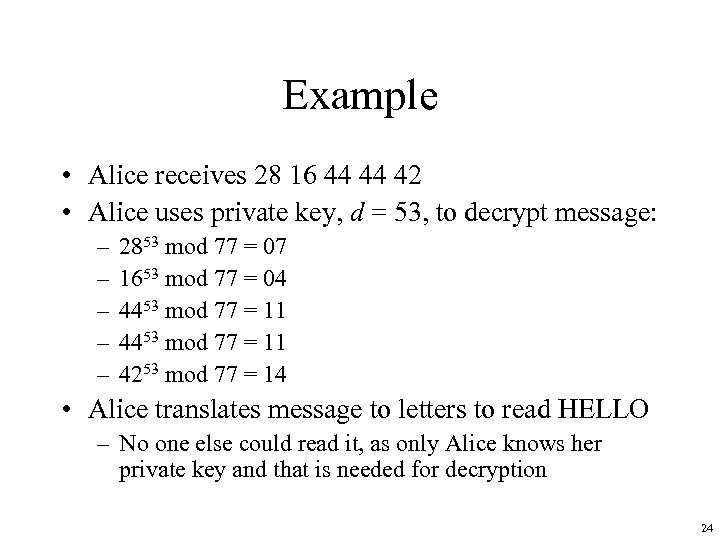Example • Alice receives 28 16 44 44 42 • Alice uses private key, d = 53, to decrypt message: – – – 2853 mod 77 = 07 1653 mod 77 = 04 4453 mod 77 = 11 4253 mod 77 = 14 • Alice translates message to letters to read HELLO – No one else could read it, as only Alice knows her private key and that is needed for decryption 24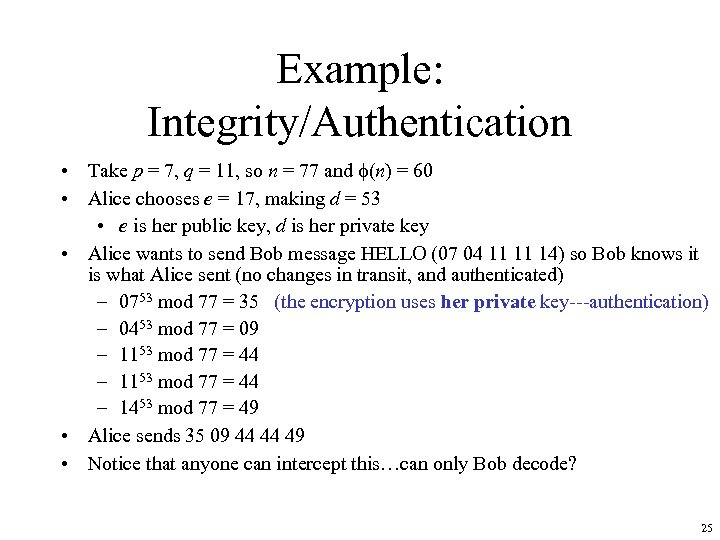Example: Integrity/Authentication • Take p = 7, q = 11, so n = 77 and (n) = 60 • Alice chooses e = 17, making d = 53 • e is her public key, d is her private key • Alice wants to send Bob message HELLO (07 04 11 11 14) so Bob knows it is what Alice sent (no changes in transit, and authenticated) – 0753 mod 77 = 35 (the encryption uses her private key---authentication) – 0453 mod 77 = 09 – 1153 mod 77 = 44 – 1453 mod 77 = 49 • Alice sends 35 09 44 44 49 • Notice that anyone can intercept this…can only Bob decode? 25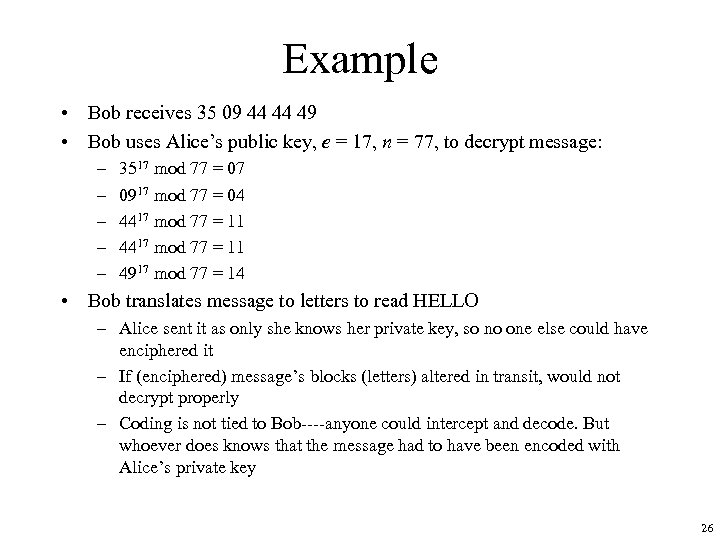Example • Bob receives 35 09 44 44 49 • Bob uses Alice’s public key, e = 17, n = 77, to decrypt message: – – – 3517 mod 77 = 07 0917 mod 77 = 04 4417 mod 77 = 11 4917 mod 77 = 14 • Bob translates message to letters to read HELLO – Alice sent it as only she knows her private key, so no one else could have enciphered it – If (enciphered) message’s blocks (letters) altered in transit, would not decrypt properly – Coding is not tied to Bob----anyone could intercept and decode. But whoever does knows that the message had to have been encoded with Alice’s private key 26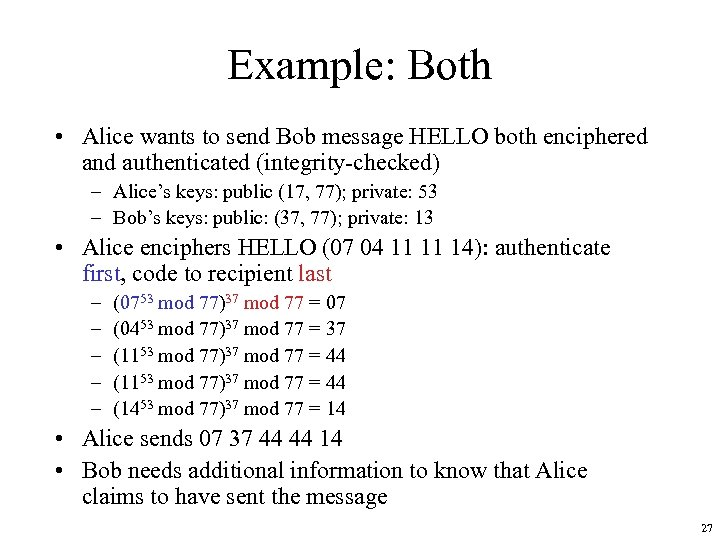Example: Both • Alice wants to send Bob message HELLO both enciphered and authenticated (integrity-checked) – Alice’s keys: public (17, 77); private: 53 – Bob’s keys: public: (37, 77); private: 13 • Alice enciphers HELLO (07 04 11 11 14): authenticate first, code to recipient last – – – (0753 mod 77)37 mod 77 = 07 (0453 mod 77)37 mod 77 = 37 (1153 mod 77)37 mod 77 = 44 (1453 mod 77)37 mod 77 = 14 • Alice sends 07 37 44 44 14 • Bob needs additional information to know that Alice claims to have sent the message 27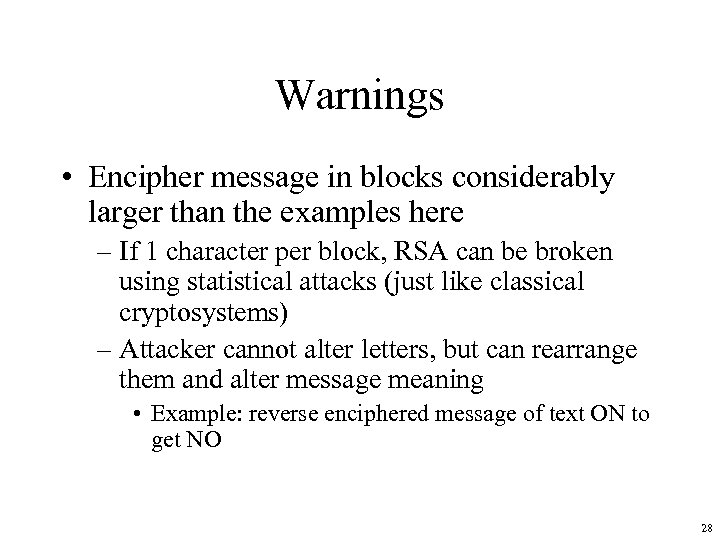Warnings • Encipher message in blocks considerably larger than the examples here – If 1 character per block, RSA can be broken using statistical attacks (just like classical cryptosystems) – Attacker cannot alter letters, but can rearrange them and alter message meaning • Example: reverse enciphered message of text ON to get NO 28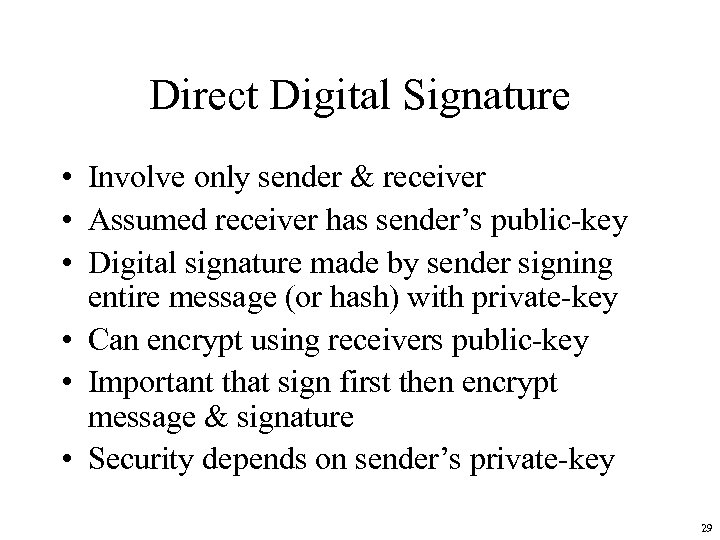Direct Digital Signature • Involve only sender & receiver • Assumed receiver has sender’s public-key • Digital signature made by sender signing entire message (or hash) with private-key • Can encrypt using receivers public-key • Important that sign first then encrypt message & signature • Security depends on sender’s private-key 29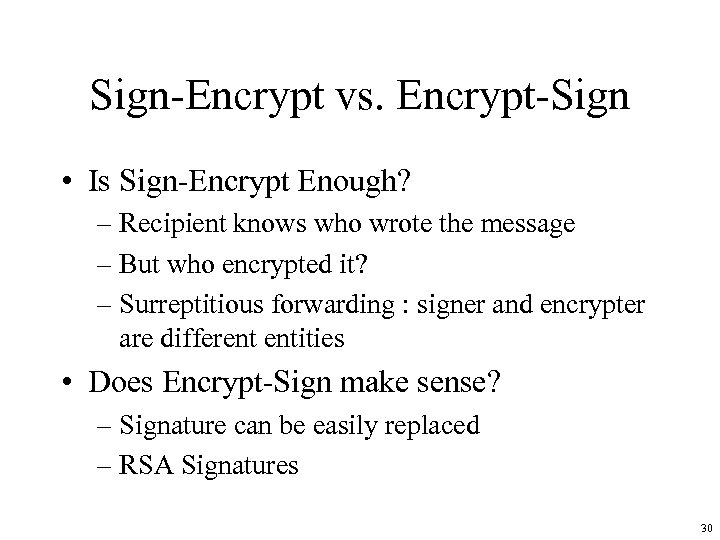Sign-Encrypt vs. Encrypt-Sign • Is Sign-Encrypt Enough? – Recipient knows who wrote the message – But who encrypted it? – Surreptitious forwarding : signer and encrypter are different entities • Does Encrypt-Sign make sense? – Signature can be easily replaced – RSA Signatures 30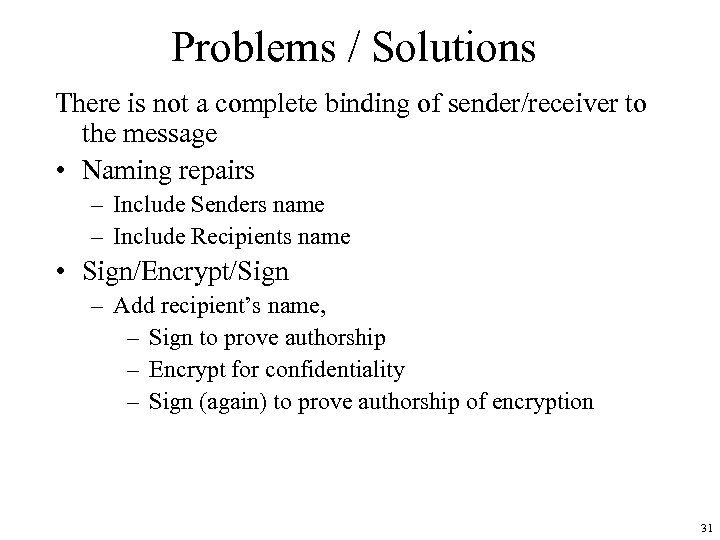Problems / Solutions There is not a complete binding of sender/receiver to the message • Naming repairs – Include Senders name – Include Recipients name • Sign/Encrypt/Sign – Add recipient’s name, – Sign to prove authorship – Encrypt for confidentiality – Sign (again) to prove authorship of encryption 31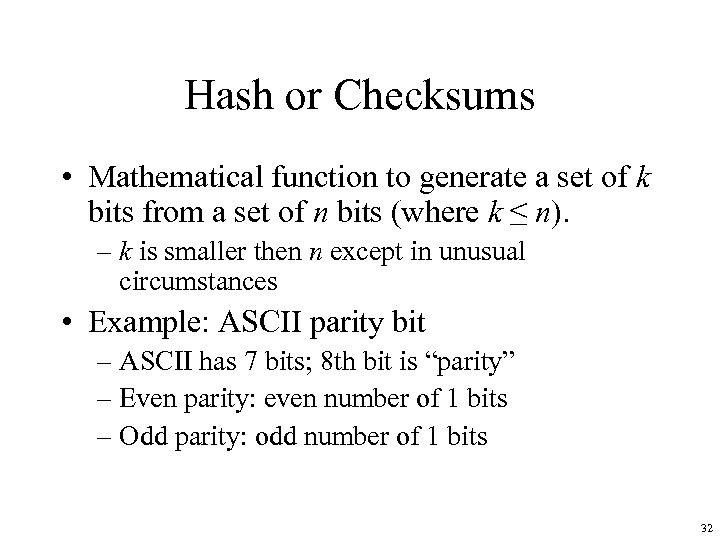Hash or Checksums • Mathematical function to generate a set of k bits from a set of n bits (where k ≤ n). – k is smaller then n except in unusual circumstances • Example: ASCII parity bit – ASCII has 7 bits; 8 th bit is “parity” – Even parity: even number of 1 bits – Odd parity: odd number of 1 bits 32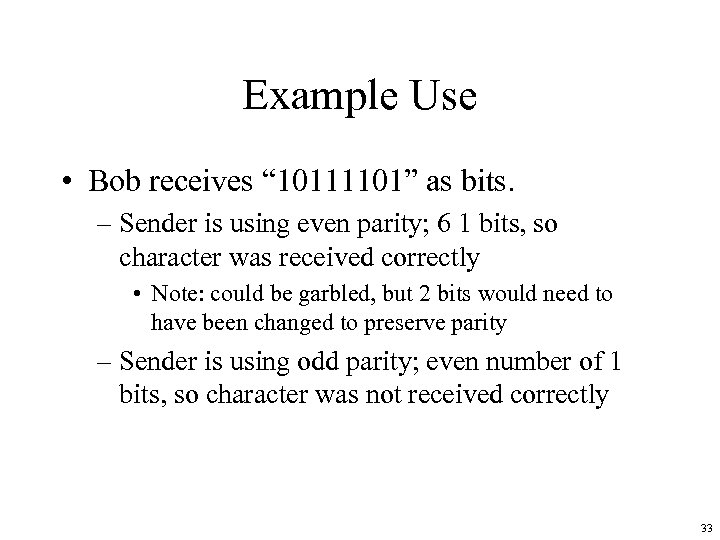Example Use • Bob receives “ 10111101” as bits. – Sender is using even parity; 6 1 bits, so character was received correctly • Note: could be garbled, but 2 bits would need to have been changed to preserve parity – Sender is using odd parity; even number of 1 bits, so character was not received correctly 33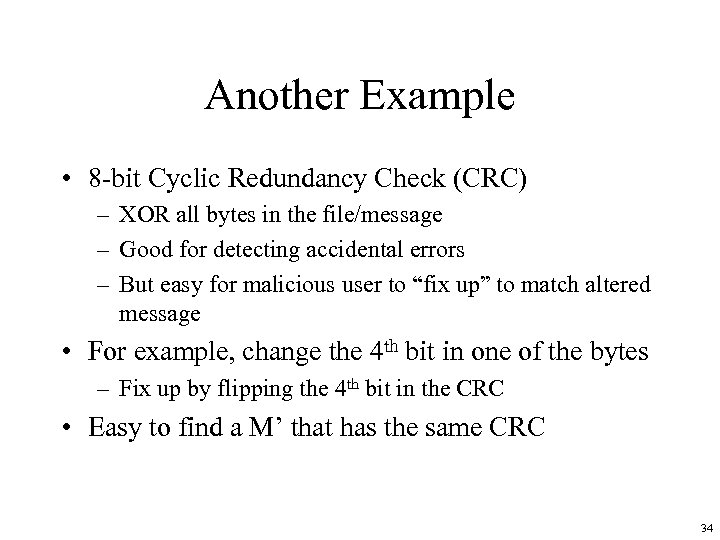Another Example • 8 -bit Cyclic Redundancy Check (CRC) – XOR all bytes in the file/message – Good for detecting accidental errors – But easy for malicious user to “fix up” to match altered message • For example, change the 4 th bit in one of the bytes – Fix up by flipping the 4 th bit in the CRC • Easy to find a M’ that has the same CRC 34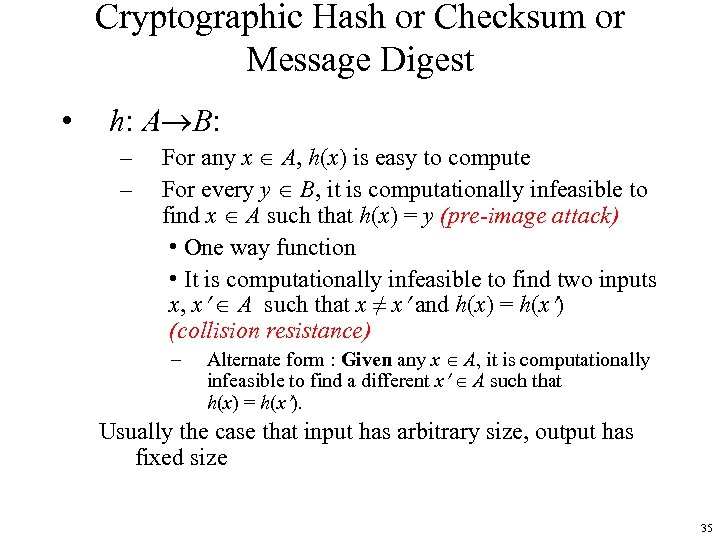Cryptographic Hash or Checksum or Message Digest • h: A B: – – For any x A, h(x) is easy to compute For every y B, it is computationally infeasible to find x A such that h(x) = y (pre-image attack) • One way function • It is computationally infeasible to find two inputs x, x A such that x ≠ x and h(x) = h(x ) (collision resistance) – Alternate form : Given any x A, it is computationally infeasible to find a different x A such that h(x) = h(x ). Usually the case that input has arbitrary size, output has fixed size 35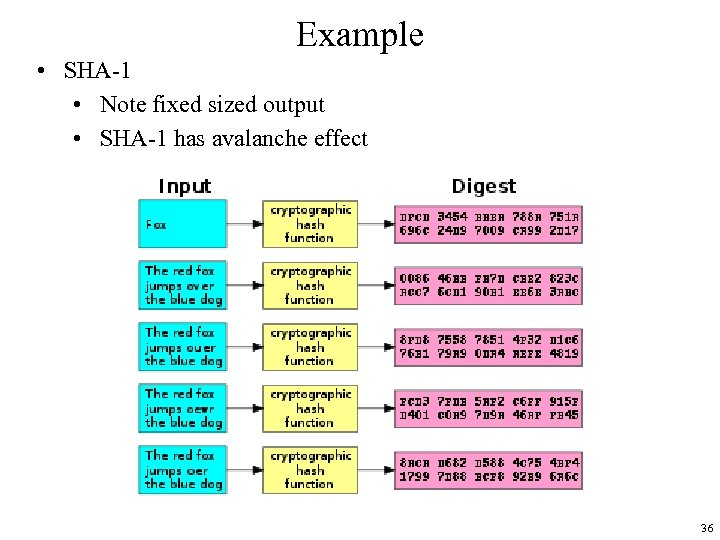Example • SHA-1 • Note fixed sized output • SHA-1 has avalanche effect 36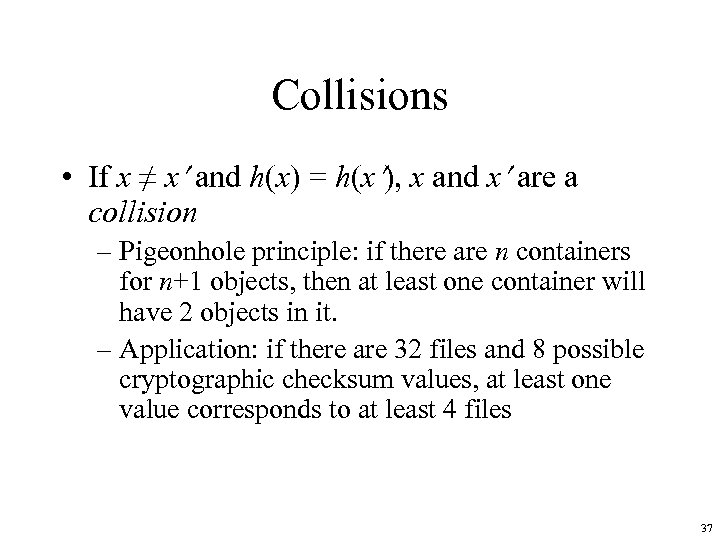Collisions • If x ≠ x and h(x) = h(x ), x and x are a collision – Pigeonhole principle: if there are n containers for n+1 objects, then at least one container will have 2 objects in it. – Application: if there are 32 files and 8 possible cryptographic checksum values, at least one value corresponds to at least 4 files 37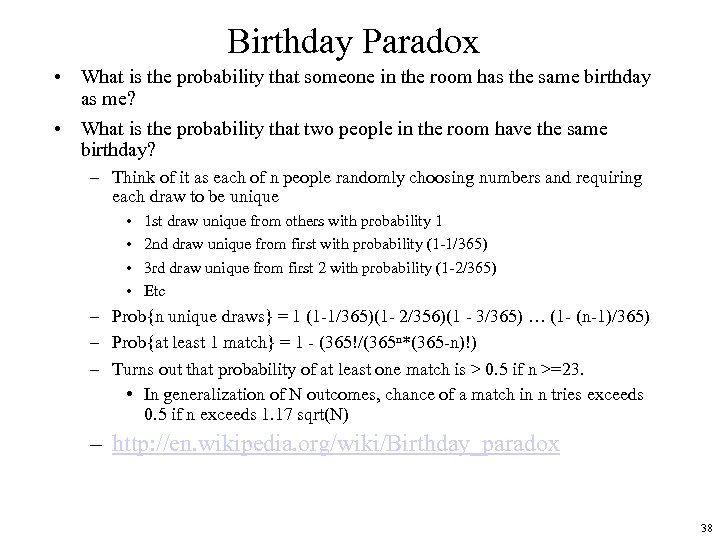Birthday Paradox • What is the probability that someone in the room has the same birthday as me? • What is the probability that two people in the room have the same birthday? – Think of it as each of n people randomly choosing numbers and requiring each draw to be unique • • 1 st draw unique from others with probability 1 2 nd draw unique from first with probability (1 -1/365) 3 rd draw unique from first 2 with probability (1 -2/365) Etc – Prob{n unique draws} = 1 (1 -1/365)(1 - 2/356)(1 - 3/365) … (1 - (n-1)/365) – Prob{at least 1 match} = 1 - (365!/(365 n*(365 -n)!) – Turns out that probability of at least one match is > 0. 5 if n >=23. • In generalization of N outcomes, chance of a match in n tries exceeds 0. 5 if n exceeds 1. 17 sqrt(N) – http: //en. wikipedia. org/wiki/Birthday_paradox 38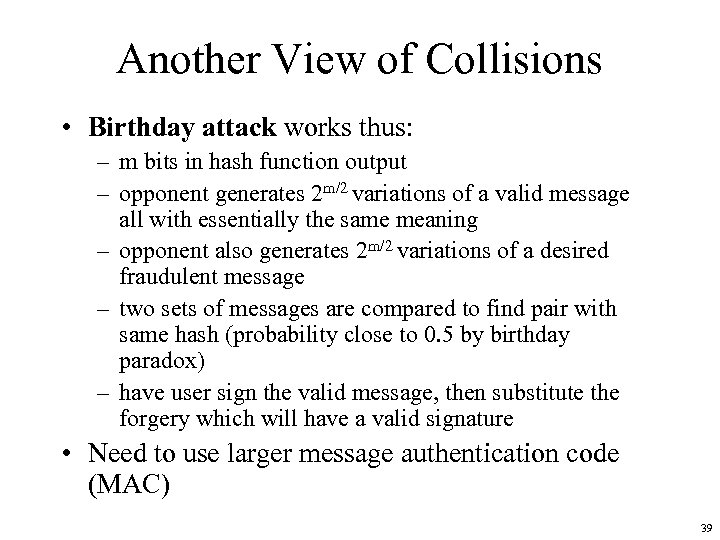Another View of Collisions • Birthday attack works thus: – m bits in hash function output – opponent generates 2 m/2 variations of a valid message all with essentially the same meaning – opponent also generates 2 m/2 variations of a desired fraudulent message – two sets of messages are compared to find pair with same hash (probability close to 0. 5 by birthday paradox) – have user sign the valid message, then substitute the forgery which will have a valid signature • Need to use larger message authentication code (MAC) 39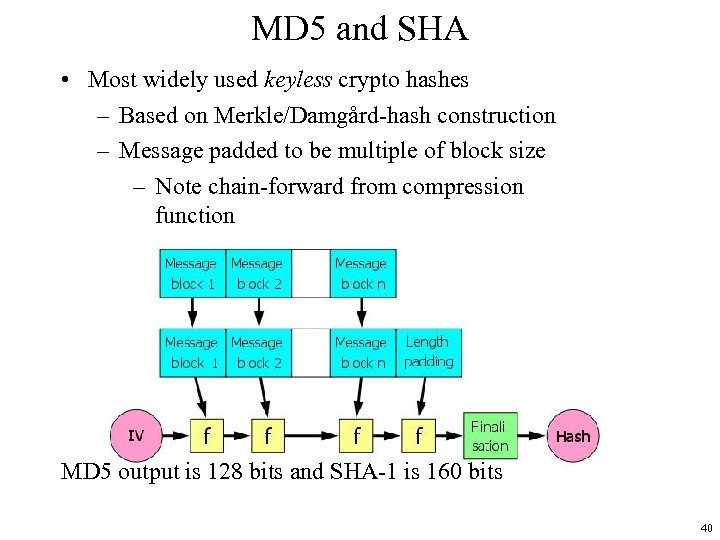MD 5 and SHA • Most widely used keyless crypto hashes – Based on Merkle/Damgård-hash construction – Message padded to be multiple of block size – Note chain-forward from compression function MD 5 output is 128 bits and SHA-1 is 160 bits 40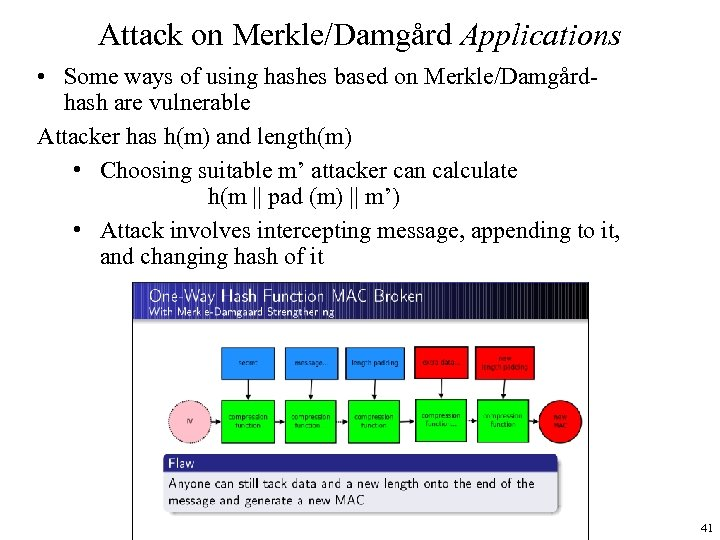Attack on Merkle/Damgård Applications • Some ways of using hashes based on Merkle/Damgårdhash are vulnerable Attacker has h(m) and length(m) • Choosing suitable m’ attacker can calculate h(m || pad (m) || m’) • Attack involves intercepting message, appending to it, and changing hash of it 41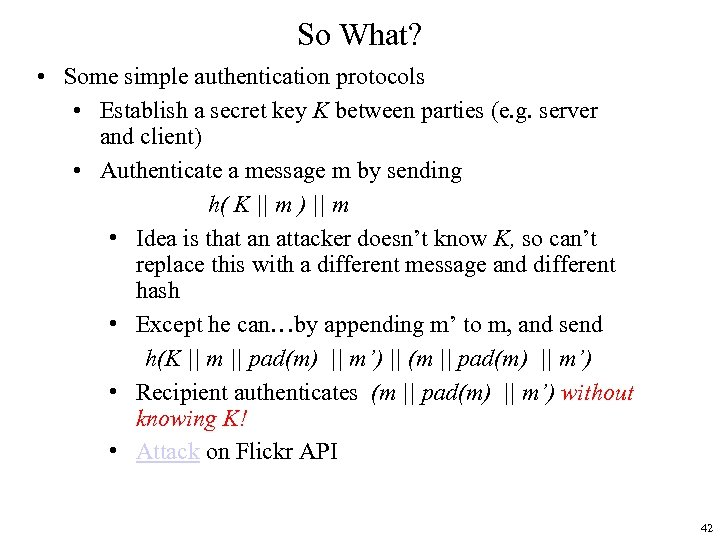So What? • Some simple authentication protocols • Establish a secret key K between parties (e. g. server and client) • Authenticate a message m by sending h( K || m ) || m • Idea is that an attacker doesn’t know K, so can’t replace this with a different message and different hash • Except he can…by appending m’ to m, and send h(K || m || pad(m) || m’) || (m || pad(m) || m’) • Recipient authenticates (m || pad(m) || m’) without knowing K! • Attack on Flickr API 42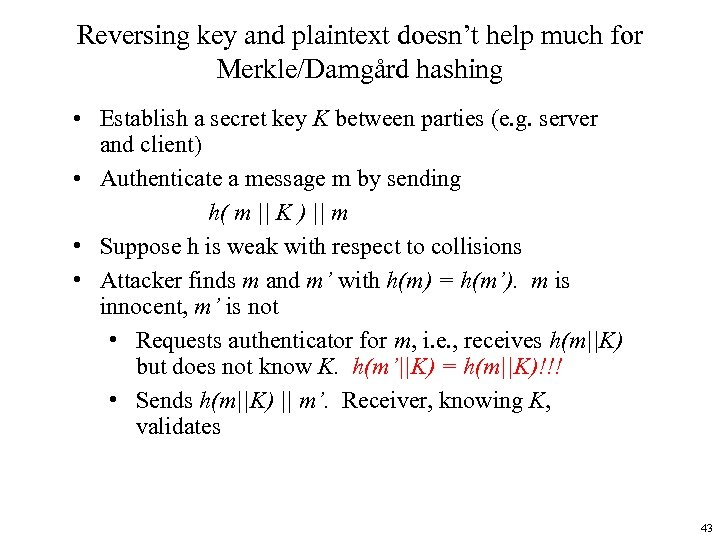Reversing key and plaintext doesn’t help much for Merkle/Damgård hashing • Establish a secret key K between parties (e. g. server and client) • Authenticate a message m by sending h( m || K ) || m • Suppose h is weak with respect to collisions • Attacker finds m and m’ with h(m) = h(m’). m is innocent, m’ is not • Requests authenticator for m, i. e. , receives h(m||K) but does not know K. h(m’||K) = h(m||K)!!! • Sends h(m||K) || m’. Receiver, knowing K, validates 43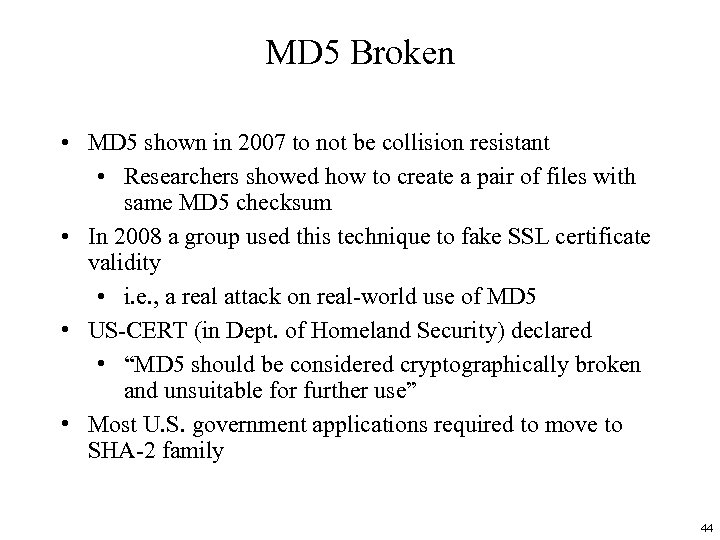MD 5 Broken • MD 5 shown in 2007 to not be collision resistant • Researchers showed how to create a pair of files with same MD 5 checksum • In 2008 a group used this technique to fake SSL certificate validity • i. e. , a real attack on real-world use of MD 5 • US-CERT (in Dept. of Homeland Security) declared • “MD 5 should be considered cryptographically broken and unsuitable for further use” • Most U. S. government applications required to move to SHA-2 family 44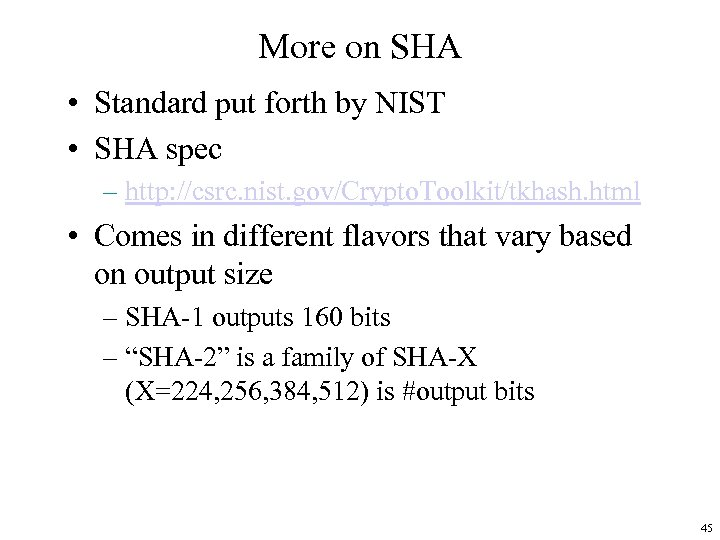More on SHA • Standard put forth by NIST • SHA spec – http: //csrc. nist. gov/Crypto. Toolkit/tkhash. html • Comes in different flavors that vary based on output size – SHA-1 outputs 160 bits – “SHA-2” is a family of SHA-X (X=224, 256, 384, 512) is #output bits 45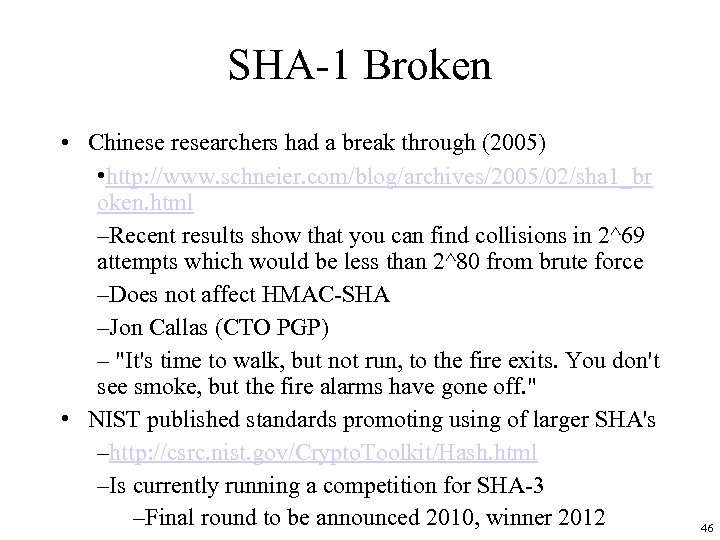SHA-1 Broken • Chinese researchers had a break through (2005) • http: //www. schneier. com/blog/archives/2005/02/sha 1_br oken. html –Recent results show that you can find collisions in 2^69 attempts which would be less than 2^80 from brute force –Does not affect HMAC-SHA –Jon Callas (CTO PGP) – "It's time to walk, but not run, to the fire exits. You don't see smoke, but the fire alarms have gone off. " • NIST published standards promoting using of larger SHA's –http: //csrc. nist. gov/Crypto. Toolkit/Hash. html –Is currently running a competition for SHA-3 –Final round to be announced 2010, winner 2012 46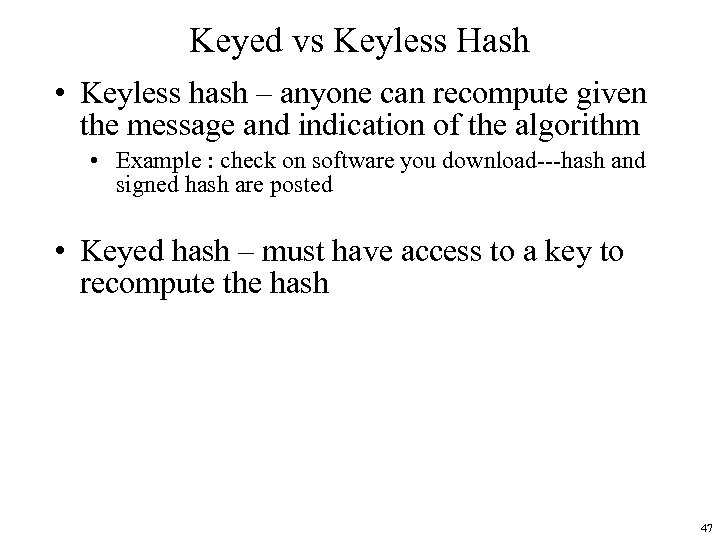Keyed vs Keyless Hash • Keyless hash – anyone can recompute given the message and indication of the algorithm • Example : check on software you download---hash and signed hash are posted • Keyed hash – must have access to a key to recompute the hash 47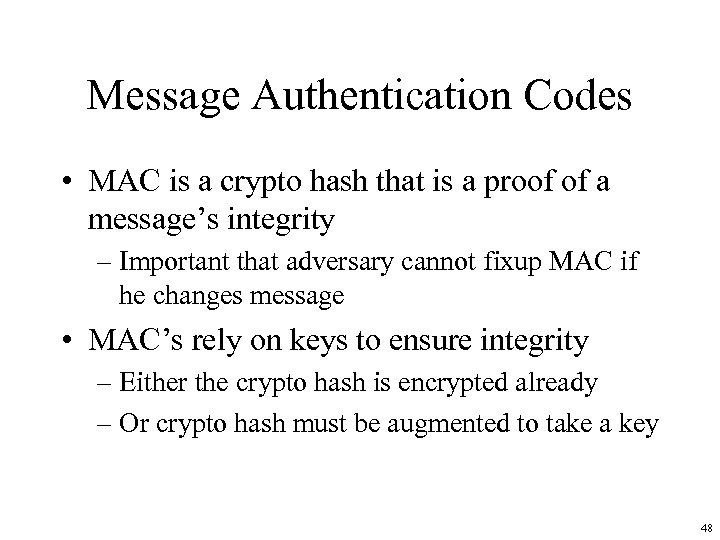Message Authentication Codes • MAC is a crypto hash that is a proof of a message’s integrity – Important that adversary cannot fixup MAC if he changes message • MAC’s rely on keys to ensure integrity – Either the crypto hash is encrypted already – Or crypto hash must be augmented to take a key 48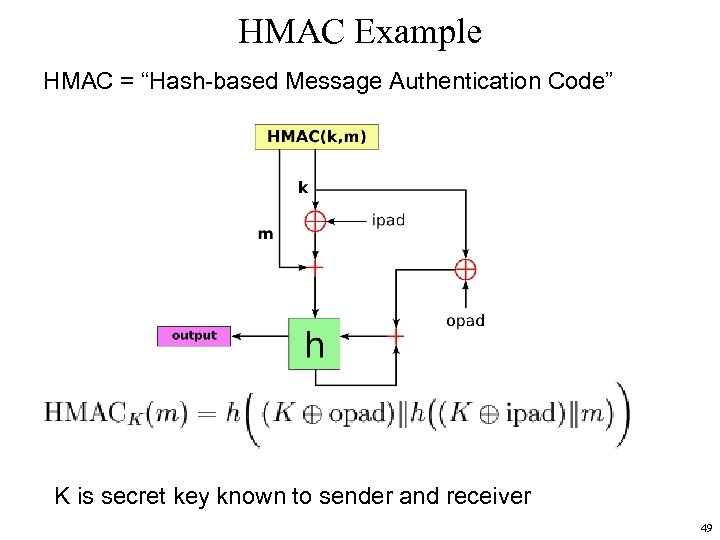HMAC Example HMAC = “Hash-based Message Authentication Code” K is secret key known to sender and receiver 49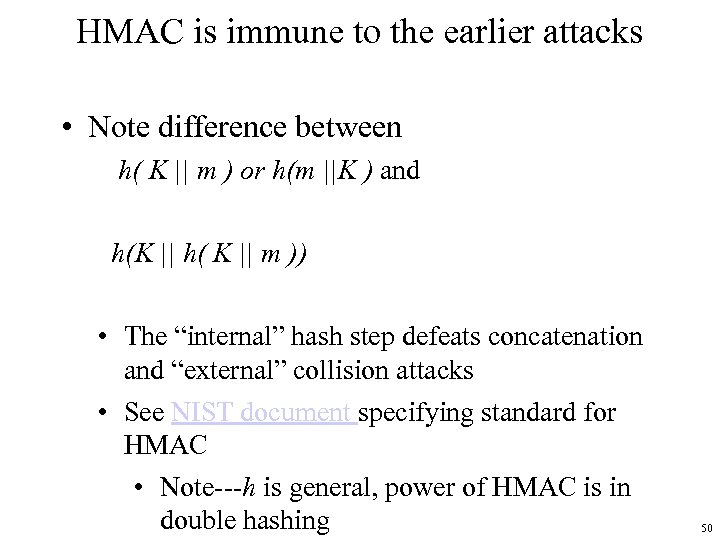HMAC is immune to the earlier attacks • Note difference between h( K || m ) or h(m ||K ) and h(K || h( K || m )) • The “internal” hash step defeats concatenation and “external” collision attacks • See NIST document specifying standard for HMAC • Note---h is general, power of HMAC is in double hashing 50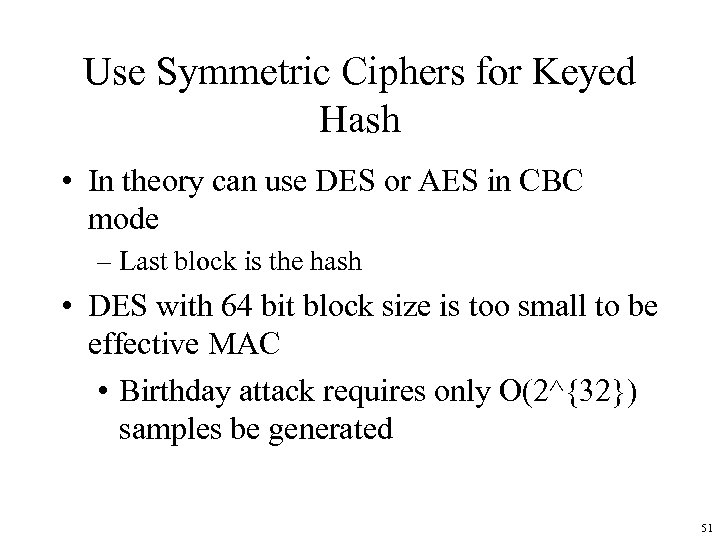Use Symmetric Ciphers for Keyed Hash • In theory can use DES or AES in CBC mode – Last block is the hash • DES with 64 bit block size is too small to be effective MAC • Birthday attack requires only O(2^{32}) samples be generated 51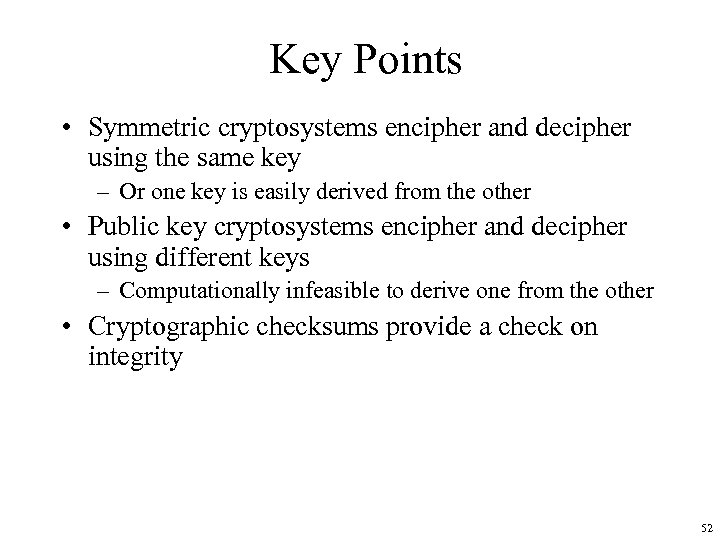Key Points • Symmetric cryptosystems encipher and decipher using the same key – Or one key is easily derived from the other • Public key cryptosystems encipher and decipher using different keys – Computationally infeasible to derive one from the other • Cryptographic checksums provide a check on integrity 52